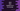# 4 different Java program to find a random value in an array## Java program to find a random value in an array:

Finding a random value from an array or from an arraylist is one common problem that we encounter. Java library class java.lang.Math provides a couple of utility methods. We can use Math.random() method to create a random number. This method can be used to create a random index for an array and that index can be used to pick a random array element.

We don’t need to install any external package as java.lang is already included.

There is one more class called java.util.Random that can be used to create random numbers as well. In this post, we will learn how to use java.lang.Math and java.util.Random to create

### Method 1: By using java.Math.random() method:

This method is defined as like below:

``public static double random()``

This is a static method and it is defined in the java.lang.Math class. This method returns a positive double value. This method returns a random value that is greater than or equal to 0.0 and less than 1.0.

On first call of this method, it creates a single new pseudorandom-number generator. This generator is used in all calls and it is not used in any other places.

We can multiply the value returned by random() with the length of the array. It will return a random value between 0.0 to array length - 1(exclusive). If we convert this value to integer, it will give a random value between 0 to array length - 1. This value can be used as the random index for the array.

Let’s write down the program:

``````class Main {
private static int getRandom(int[] arr) {
int randomIndex = (int) (Math.random() * arr.length);

return arr[randomIndex];
}

public static void main(String[] args) {
int[] givenArray = new int[]{10, 20, 30, 40, 50, 60, 70, 80, 90};

System.out.println("Random value: " + getRandom(givenArray));
}
}``````

Here,

• getRandom method is used to get a random value from an array.
• It takes an array as the parameter and returns a random value of the array. We are using integer array for this example but you can use any type.
• It multiplies the value returned by the Math.random() method with array length and cast that value to integer to create a random index. Then, it returns the value pointed by this index.

If you run this program, it will print a random value of givenArray.

``Random value: 70``

### Method 2: By using java.util.Random:

java.util.Random class instance can be used to create random values. This class can be instantiate in two ways:

``````Random()

Random(long seed)``````

If we create two instances with the same seed, and same sequence of methods are called on these instances, it will return identical sequence of numbers.

For this example, we will use `System.currentTimeMillis()` to create a random seed for the initialization. It will create different values even if you use multiple instances of Random.

We will use the below method to create a random index:

``int nextInt(int bound)``

Array length will be passed as bound. It returns a random integer between 0(inclusive) to bound(exclusive).

``````import java.util.Random;

class Main {
private static int getRandom(int[] arr) {
int randomIndex = new Random(System.currentTimeMillis()).nextInt(arr.length);

return arr[randomIndex];
}

public static void main(String[] args) {
int[] givenArray = new int[]{10, 20, 30, 40, 50, 60, 70, 80, 90};

System.out.println("Random value: " + getRandom(givenArray));
}
}``````

### Method 3: By using java.util.concurrent.ThreadLocalRandom:

ThreadLocalRandom class extends Random class. It is another random number generator isolated to the current thread.

``````import java.util.concurrent.ThreadLocalRandom;

class Main {
private static int getRandom(int[] arr) {

return arr[randomIndex];
}

public static void main(String[] args) {
int[] givenArray = new int[]{10, 20, 30, 40, 50, 60, 70, 80, 90};

System.out.println("Random value: " + getRandom(givenArray));
}
}``````

It will give similar output.

### Method 4: By using SecureRandom:

None of the above methods are cryptographically secure. We can use `java.security.SecureRandom` class instance to create cryptographically strong random numbers. This method is also extends from the Random class and we can create instances with or without providing a seed value:

``````SecureRandom()

SecureRandom(byte[] seed)``````
``````import java.security.SecureRandom;

class Main {
private static int getRandom(int[] arr) {
byte[] bytes = new byte;
SecureRandom random = new SecureRandom(bytes);

int randomIndex = random.nextInt(arr.length);

return arr[randomIndex];
}

public static void main(String[] args) {
int[] givenArray = new int[]{10, 20, 30, 40, 50, 60, 70, 80, 90};

System.out.println("Random value: " + getRandom(givenArray));
}
}``````